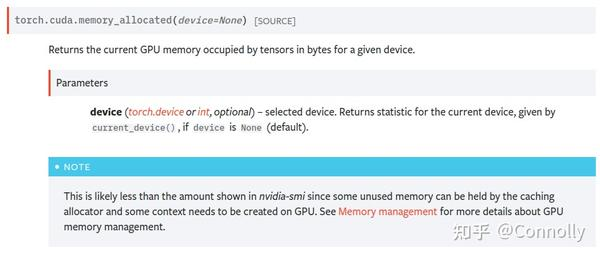• 问答
• 技术
• 实践
• 资源
PyTorch 显存机制分析

OS:Ubuntu18.04
python:3.7.4
PyTorch:1.9.1
GPU:V100

1 理论知识
1.1 深度学习训练过程
1.2 前向传播
1.3 后向传播（反向传播）
1.4 梯度更新
2 显存分析方法与Torch机制
2.1 分析方法
2.2 Torch显存分配机制
2.3 Torch显存释放机制
3 训练过程显存分析
3.1 模型的定义
3.2 前向传播过程
3.3 后向传播过程
3.4 参数更新

1 理论知识

1.1 深度学习训练过程

1. 模型定义：定义了模型的网络结构，产生模型参数；

while(你想训练):

1. 前向传播：执行模型的前向传播，产生中间激活值；
2. 后向传播：执行模型的后向传播，产生梯度；
3. 梯度更新：执行模型参数的更新，第一次执行的时候产生优化器状态。

1.2 前向传播

$Y=W^TX+b$ ，

1.3 后向传播（反向传播）

$$\delta W= out_{diff} X^T$$

$$\delta b = out_{diff}$$

$$in_{diff}=W^T Y$$

1.4 梯度更新

$$W-=learningrate * \delta W$$

$$b-=learningrate*\delta b$$

2 显存分析方法与Torch机制

2.1 分析方法

(1) No Nvidia-smi

(2) torch.cuda is all you need.

memory_allocated+memory_reserved就等于nvidia-smi中的值啦。

{非~常~好~用|chao da sheng}3 训练过程显存分析

import torch

model = torch.nn.Linear(1024,1024, bias=False).cuda()
inputs = torch.tensor([1.0]*1024).cuda() # shape = (1024)
outputs = model(inputs) # shape = (1024)
loss = sum(outputs) # shape = (1)
loss.backward()
optimizer.step()

3.1 模型的定义

import torch

model = torch.nn.Linear(1024,1024, bias=False).cuda()
print(torch.cuda.memory_allocated())

3.2 前向传播过程

inputs = torch.tensor([1.0]*1024).cuda() # shape = (1024)  memory + 4096
outputs = model(inputs) # memory + 4096

3.3 后向传播过程

loss = sum(outputs) # memory + 512(torch cuda分配最小单位)
temp = torch.cuda.memory_allocated()
loss.backward()
print(torch.cuda.memory_allocated() - temp) # 第一次增加4194304

Note：由于这个中间激活值被赋给了outputs，所以后面在后向传播的时候会发现，这个outputs的显存没有被释放掉。但是当层数变深的时候，就能明显看到变化了。

import torch

# 模型初始化
linear1 = torch.nn.Linear(1024,1024, bias=False).cuda() # + 4194304
print(torch.cuda.memory_allocated())
linear2 = torch.nn.Linear(1024, 1, bias=False).cuda() # + 4096
print(torch.cuda.memory_allocated())

# 输入定义
inputs = torch.tensor([[1.0]*1024]*1024).cuda() # shape = (1024,1024) # + 4194304
print(torch.cuda.memory_allocated())

# 前向传播
loss = sum(linear2(linear1(inputs))) # shape = (1) # memory + 4194304 + 512
print(torch.cuda.memory_allocated())

# 后向传播
loss.backward() # memory - 4194304 + 4194304 + 4096
print(torch.cuda.memory_allocated())

# 再来一次~
loss = sum(linear2(linear1(inputs))) # shape = (1) # memory + 4194304  (512没了，因为loss的ref还在)
print(torch.cuda.memory_allocated())
loss.backward() # memory - 4194304
print(torch.cuda.memory_allocated())

3.4 参数更新

optimizer.step() # 第一次增加8388608，第二次就不增不减了哦

•1
•0
•1409收藏

• 35

关注
• 106

获赞
• 23

精选文章

• 医学图像 图像分类

• 黑人的专栏

• 636
• 73
• 65
• 80
• 65